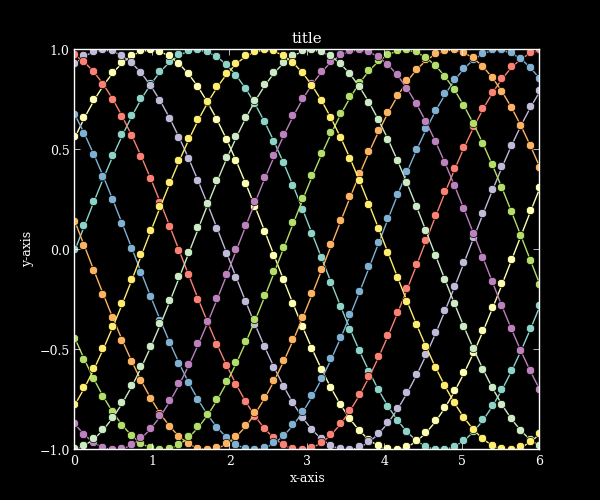# Dark backgrounds¶

A key feature of mpltools is the ability to set “styles”—essentially, stylesheets that are similar to matplotlibrc files. This example demonstrates the “dark_background” style, which uses white for elements that are typically black (text, borders, etc). Note, however, that not all plot elements default to colors defined by an rc parameter.

import numpy as np
import matplotlib.pyplot as plt

from mpltools import style
style.use('dark_background')

L = 6
x = np.linspace(0, L)
ncolors = len(plt.rcParams['axes.color_cycle'])
shift = np.linspace(0, L, ncolors, endpoint=False)
for s in shift:
plt.plot(x, np.sin(x + s), 'o-')
plt.xlabel('x-axis')
plt.ylabel('y-axis')
plt.title('title')

plt.show()